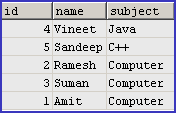Tutorials

# MySQL random

In MySQL we can get the random records by using the method RAND() of MySQL. There are two versions of RAND() function : first RAND() and second is RAND(X).

In MySQL we can get the random records by using the method RAND() of MySQL. There are two versions of RAND() function : first RAND() and second is RAND(X).

# MySQL random

In MySQL we can get the random records by using the method RAND() of MySQL. There are two versions of RAND() function : first RAND() and second is RAND(X). If simple RAND() is used then it returns the floating value in between 0 to 1.0 (including both 0 and 1) and if any integer argument is passed, it takes X as it's seed value and query results a repeatable sequence of column values.

Here in the following example we will describe how you can use RAND()  and RAND(X) function to fetch records from the database.

Firstly we will explain the use of RAND(), that's why we have created a table "mca". Here is the full table structure and query for creating the table as given below :

 Query CREATE TABLE `mca` ( `id` bigint(11) NOT NULL auto_increment, `name` varchar(255) default NULL, `subject` varchar(255) default NULL, PRIMARY KEY (`id`) ) ; /*Data for the table `mca` */ insert into `mca`(`id`,`name`,`subject`) values (1,'Amit','Computer'), (2,'Ramesh','Computer '),(3,'Suman','Computer'), (4,'Vineet','Java'),(5,'Sandeep','C++'); OutputNow we can execute the update query on the table "mca"

 Query SELECT * FROM mca ORDER BY RAND(); OutputYou can see from the output that it selects the row database in the random order via RAND() function.

In the example below, We have created a variable Number in which generated random number is being taken and thereafter we can show the result of it. Numbers generated are in the random order which means that they lack any pattern.

 ```mysql> set @Number = RAND(); Query OK, 0 rows affected (0.00 sec)``` ```mysql> SELECT @Number as Number; +------------------+ | Number | +------------------+ | 0.44307944592375 | +------------------+ 1 row in set (0.00 sec) ``` ```mysql> set @Number = RAND(); Query OK, 0 rows affected (0.00 sec) mysql> SELECT @Number as Number; +------------------+ | Number | +------------------+ | 0.63046264893418 | +------------------+ 1 row in set (0.00 sec) ```

Now we are going to explain you use of RAND(X) with the simple example.

 ```mysql> set @Number = RAND(1); Query OK, 0 rows affected (0.00 sec)``` ```mysql> SELECT @Number as Number; +------------------+ | Number | +------------------+ | 0.40540353712198 | +------------------+ 1 row in set (0.00 sec)``` ```mysql> set @Number = RAND(1); Query OK, 0 rows affected (0.00 sec)``` ```mysql> SELECT @Number as Number; +------------------+ | Number | +------------------+ | 0.40540353712198 | +------------------+ 1 row in set (0.00 sec)```

You can see that it generates the same number since we have provided seed value in the RAND() function.